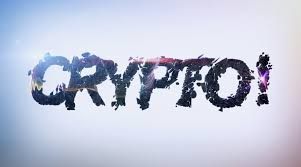# Questions tagged as ['quantum-cryptography']

Quantum cryptography describes the use of quantum mechanical effects to perform cryptographic tasks. This is not to be confused with quantum computing or cryptanalysis techniques such as Shor's algorithm.
Score: 0QKD measuring qubit with wrong basesI'm trying to end the research work for my master thesis about BB84 QKD (and QBC) and a basic problem of quantum mechanics is blocking me.

I'm trying to do a probability calculus of the action of measuring a qubit in a wrong bases. In bibliography, I've always found the statement:

When Bob chooses the wrong bases for measuring a qubit then the result will be completely random.

But what exactly d ...

Score: 4A question about performing quantum computations on uniform superpositionsLet us consider the following situation. Let $$U_f$$ be a gate computing $$f$$ mapping $$\{0,1\}^n$$ to $$\{0,1\}^n$$. That is, $$U_f\left\vert x,0^n\right\rangle=\left\vert x,f(x)\right\rangle$$. Let $$\left\vert\phi\right\rangle$$ be the uniform superposition on $$\{0,1\}^n$$. By performing $$U_f$$ on $$\left\vert\phi\right\rangle\left\vert0^n\right\rangle$$, we have $$\left\vert\phi'\right\rangle=\sum_{x\in\{0,1\}^n ...$$

Score: 5What are the misconceptions of IBM's CEO Arvind Krishna talk on the "Axios on HBO" about the quantum computingIBM CEO Arvind made a talk in HBO's Axios program. It seems that there are misconceptions/misleading/flaws in reasoning etc.

What are those!

Some of the details of the speech is given as;

IBM says its new Eagle processor can handle 127 qubits, a measure of quantum computing power. In topping 100 qubits, IBM says it has reached a milestone that allows quantum to surpass the power of a traditional comp ...

Score: 24What does the work "An Efficient Quantum Algorithm for Lattice Problems Achieving Subexponential Approximation Factor" mean?In An Efficient Quantum Algorithm for Lattice Problems Achieving Subexponential Approximation Factor, the author claims they give a polynomial-time quantum algorithm for solving the Bounded Distance Decoding problem with a subexponential approximation factor on a class of integer lattices. What does this result mean? Will it imply the insecurity of lattice cryptography? Is it as important as quan ...

Score: 4In quantum cryptography, why can a qubit can be both 0 and 1 at the same?The texts below are from some tutorial, and it says (in bold format below) a qubit can be both 0 and 1 at the same time. It sounds very strange to me, can you please explain why?

Quantum cryptography utilises the physics of photons (light energy according to the formula E = hf) and their physical quantum properties to produce a virtually unbreakable encryption system. This helps protect the security of  ...